# Shift operator

Jump to: navigation, search

An operator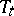that depends on a parameterand acts in a setof mappings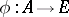(whereis an Abelian semi-group andis a set) in accordance with the formula(is also called the operator of shift by). The semi-groupis often taken to beor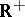(thenis a shift in some space of functions of a real variable),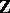or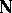(thenis a shift in some space of sequences). The setand the corresponding setare usually endowed with a certain structure (of a vector, topological vector, normed, metric, or probability space).

A shift operator is used, in particular, in the theory of dynamical systems (see Shift dynamical system; Bernoulli automorphism). Also used is the terminology "shift operator along the trajectories of differential equations" (see Cauchy operator).

#### Comments

The discrete dynamical systems generated by shift operators on sequence spaces are often easy to analyze. They are of great importance in dynamical systems theory, owing to the Smale–Birkhoff theorem: A discrete-time dynamical system containing a homoclinic point at which the stable and unstable manifolds interact transversely, must contain a compact invariant set on which the dynamics is isomorphic to a certain type of shift in which periodic orbits are dense. This is the best-known method for demonstrating deterministic chaos ([a1], [a2]).